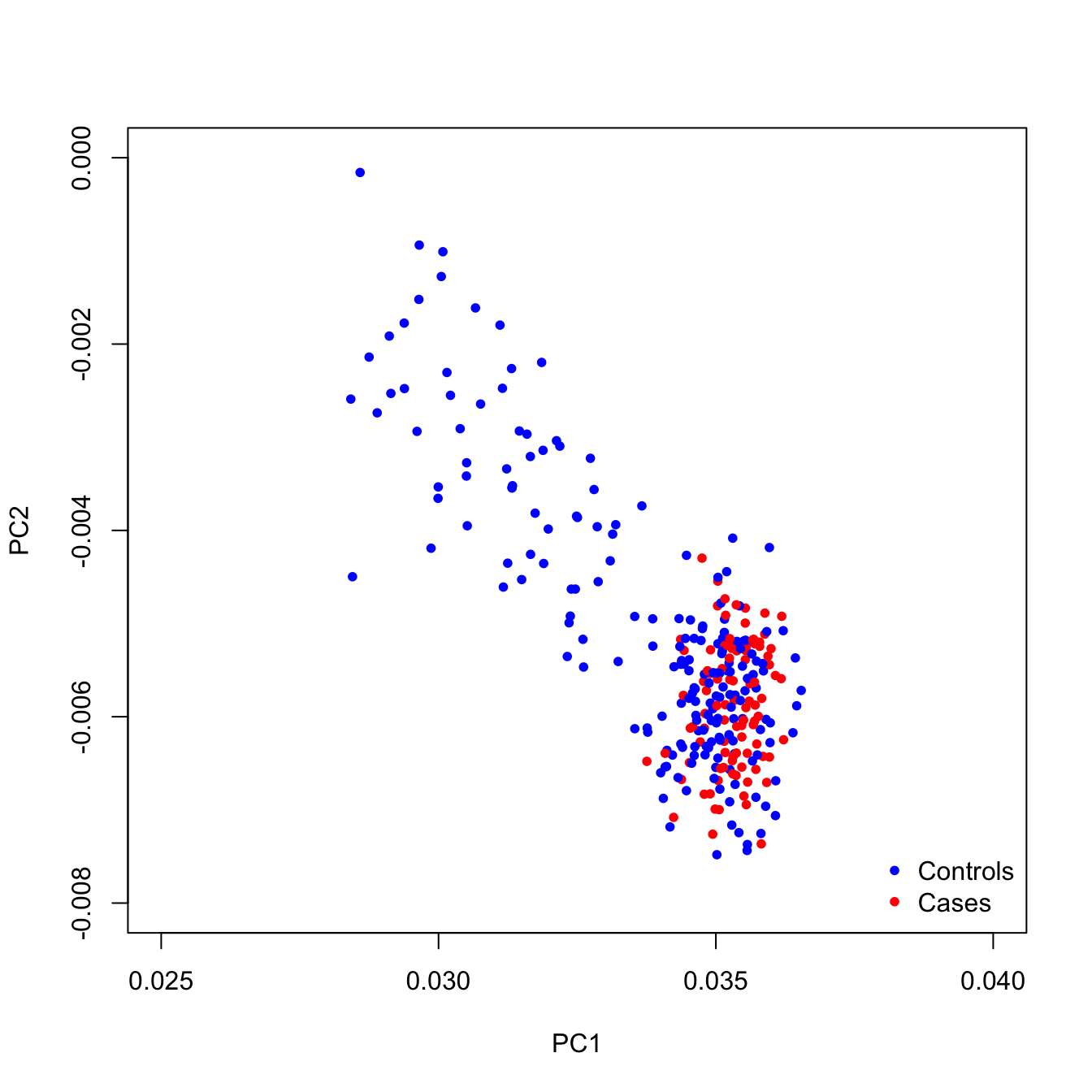## Description

PCAmatchR matches cases to controls based on genotype principal components (PC). In order to produce more genetically similar matches, a weighted Mahalanobis distance metric (Kidd et al. (1987)) is used to determine matches. Weights are equal to the percent variance explained by each PC.

## Installation

The release version of PCAmatchR can be installed from CRAN:

``install.packages("PCAmatchR")``

The development version of the PCAmatchR package can be installed from the GitHub repository by using the devtools package:

``devtools::install_github("machiela-lab/PCAmatchR")``

PCAmatchR depends on the optmatch package which must be manually installed from CRAN:

``install.packages("optmatch")``

Following installation, attach the PCAmatchR and optmatch packages with:

``````library(PCAmatchR)
library(optmatch)``````

## Usage

Here we perform a hypothetical example of case-control matching using the Phase 3 data release of 1000 Genomes Project, which contains genotype data from 2,504 individuals from 26 distinct populations (available at https://www.cog-genomics.org/plink/2.0/resources).

### Available Data

Within PCAmatchR, we include sample data containing information about population, gender, and the first 20 principal components and eigenvalues calculated from 2,504 individuals in the Phase 3 data release of the 1000 Genomes Project. The example principal component analysis was conducted with PLINK using a set of ancestry informative SNPs (Yu et al. (2008)). The data files are contained within:

• `PCs_1000G`, A sample dataset containing information about population, gender, and the first 20 principal components calculated from 2,504 individuals in the Phase 3 data release of the 1000 Genomes Project.

• `eigenvalues_1000G`, A sample dataset containing the first 20 eigenvalues calculated from 2,504 individuals in the Phase 3 data release of the 1000 Genomes Project.

• `eigenvalues_all_1000G`, A sample dataset containing all eigenvalues calculated from 2,504 individuals in the Phase 3 data release of the 1000 Genomes Project.

``````# Load required packages

# Create PC data frame
pcs<- as.data.frame(PCs_1000G[,c(1,5:24)])

# Create eigenvalues vector
eigen_vals<- c(eigenvalues_1000G)\$eigen_values

# Create full eigenvalues vector
all_eigen_vals<- c(eigenvalues_all_1000G)\$eigen_values``````

### Case and Control Populations

For this example analysis, we select individuals from the ESN (Esan in Nigeria) population as cases (N=99), while all remaining samples are used as the control population (N=2,405):

``````covariate_data<- as.data.frame(PCs_1000G[,1:4])
covariate_data\$case <- ifelse(covariate_data\$pop=="ESN", c(1), c(0))``````

### Case-Control Matching

Matching is performed using `match_maker`. Within this example, cases and controls are 1:2 matched on the first 20 PCs:

``````match_maker_output<- match_maker(PC = pcs,
eigen_value = eigen_vals,
data = covariate_data,
ids = c("sample"),
case_control="case",
num_controls = 2,
eigen_sum = sum(all_eigen_vals))``````

Derived matches are contained within the `matches` object. The weighted Mahalanobis distance metric between case and control pairs is detailed within the `match_distance` variable:

``````PCA_matches<- match_maker_output\$matches
PCA_matches\$match_distance``````

### Case-Control Matching Visualization

The distance between matches can be visualized using `plot_maker`:

``````plot_maker(data=match_maker_output,
x_var="PC1",
y_var="PC2",
case_control="case",
line=F,
xlim = c(0.025,0.04),
ylim = c(-0.008,0.00))``````The function further allows for connections between matches:

``````plot_maker(data=match_maker_output,
x_var="PC1",
y_var="PC2",
case_control="case",
line=T,
xlim = c(0.025,0.04),
ylim = c(-0.008,0.00))``````